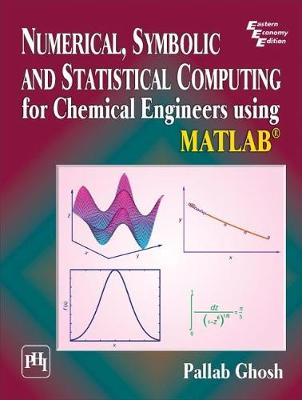•# Numerical, Symbolic and Statistical Computing for Chemical Engineers using Matlab ® (Paperback)

(author)
£25.50
Paperback 416 Pages / Published: 30/10/2018
• In stock
• Free UK delivery

Usually dispatched within 24 hours

Numerical, analytical and statistical computations are routine affairs for chemical engineers. They usually prefer a single software to solve their computational problems, and at present, MATLAB has emerged as a powerful computational language, which is preferably used for this purpose, due to its built-in functions and toolboxes.

Considering the needs and convenience of the students, the author has made an attempt to write this book, which explains the various concepts of MATLAB in a systematic way and makes its readers proficient in using MATLAB for computing. It mainly focuses on the applications of MATLAB, rather than its use in programming basic numerical algorithms.

Commencing with the introduction to MATLAB, the text covers vector and matrix computations, solution of linear and non-linear equations, differentiation and integration, and solution of ordinary and partial differential equations. Next, analytical computations using the Symbolic Math Toolbox and statistical computations using the Statistics and Machine Learning Toolbox are explained. Finally, the book describes various curve fitting techniques using the Curve Fitting Toolbox. Inclusion of all these advanced-level topics in the book stands it out from the rest.

Primarily intended for the undergraduate students of chemical engineering, the book is also of immense use to the postgraduate students, chemical engineers and scientists.

KEY FEATURES:Numerous worked-out examples to enable the readers understand the steps involved in solving the chemical engineering problems MATLAB codes to explain the computational techniquesSeveral snapshots to help the readers understand the step-by-step procedures of using the toolboxesChapter-end exercises, including short-answer questions and numerical problemsAppendix comprising the definitions of some important and special matricesSupplemented with Solutions Manual containing complete detailed solutions to the unsolved problemsAccessibility of selected colour figures (including screenshots and results/outputs of the programs) cited in the text at www.phindia.com/Pallab_Ghosh.

Publisher: PHI Learning
ISBN: 9789387472518
Number of pages: 416
Dimensions: 229 x 152 mm